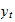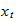StatLect

# Training, validation and test samples

In order to avoid overfitting and produce unbiased estimates of the risk of our predictive models, we usually randomly split our data into:

• training sample;

• validation sample;

• test sample.## Training sample

We use the training sample to:

• set the parameters of the predictive models ("train the models" in machine learning parlance).

Example: estimation of the coefficients of several different linear regression models.

## Validation sample

We use the validation sample to:

• choose the best model among the different models trained on the training sample.

How do we perform the choice? By selecting the model that has the lowest empirical risk on the validation sample.

Example: choice between a more parsimonious regression model and a less parsimonious one, both estimated on the training sample. We choose the model that has the lowest mean squared prediction error on the validation sample.

## Test sample

We use the test sample to:

• obtain an unbiased estimate of the risk of the model selected in the validation step.

Why is this step necessary? Because model selection produces effects similar to parameter estimation: it makes the empirical risk of the best model on the validation sample a downward-biased estimate of its true risk. As a matter of fact, model selection can often be framed as an (hyper-)parameter optimization problem. In other words, model selection causes some overfitting.

Remarks:

• In serious forecasting competitions (e.g., on Kaggle), prediction models are submitted and then tested by someone else on data that participants cannot see (in some cases, on data that does not even exist when the submission is performed).

• Think about what happens if you find that the performance on the test sample is not good. If what happens is that you start again and re-use the test sample, then think again: you might want to split the test sample in two parts and use one for intermediate testing (Train-Validation-Test1-Test2).

## Warning

In the machine learning literature, the term "validation sample" is sometimes used with a different meaning:

• what we called above training and validation samples are collectively called a training sample (because model selection is seen as just another form of training);

• what we called above a test sample is called a validation sample.

Indeed, the practice of using a test sample to estimate the risk of a predictive model is called (holdout) cross validation.

## How to split

There is no universally accepted rule for deciding what proportions of data should be allocated to the three samples (train, validation, test).

The general criterion is to have enough data in the validation and test samples to reliably estimate the risk of the predictive models.

Some popular choices are: 60-20-20, 70-15-15, 80-10-10.

Remember: producing reliable estimates of the true risk of our predictive models is more important than anything else!

## Example: predicting inflation

We now learn how to do train-val-test splits with Python and the scikit-learn package.

### Import the data

We are going to use a data set where:

• the outputis month-on-month HICP inflation in the euro area;

• the input vectorincludes monthly dummies (to capture seasonality) and more than 100 real-time macroeconomic variables.

``````import numpy as np # Numpy is a Matlab-like package for array manipulation and linear algebra
import pandas as pd # Pandas is a data-analysis and table-manipulation tool

try:
except:
y = y.values # Transform y into a numpy array

# Print some information about the output variable
print('Class and dimension of output variable:')
print(type(y))
print(y.shape)``````

The output is:

``````Class and dimension of output variable:
class 'numpy.ndarray'
(270, 1)``````
``````# Load the input variables with pandas
try:
except:
x = x.values

# Print some information about the input variables
print('Class and dimension of input variables:')
print(type(x))
print(x.shape)``````

The output is:

``````Class and dimension of input variables:
class 'numpy.ndarray'
(270, 113)``````

### Use scikit-learn to split into train-val-test (60-20-20)

As scikit-learn only performs splits into two sub-samples, we first create the training sample, and then we split the remaining observations into validation and test.

``````# Import the function that performs sample splits from scikit-learn
from sklearn.model_selection import train_test_split

# Create the training sample
x_train, x_val_test, y_train, y_val_test
= train_test_split(x, y, test_size=0.4, random_state=1)

# Split the remaining observations into validation and test
x_val, x_test, y_val, y_test
= train_test_split(x_val_test, y_val_test, test_size=0.5, random_state=1)

# Print the numerosities of the three samples
print(x_train.shape, x_val.shape, x_test.shape)``````

The output is:

``162 54 54``

### Estimate and test a linear regression with all inputs

Here we show that a linear regression model with lots of parameters, estimated by ordinary least squares, overfits on the training set and has a disappointing performance on the validation set.

We are not yet using the test set because we are going to try other models and then pick the best one.

``````# Import functions from scikit-learn
from sklearn import linear_model # Linear regression
from sklearn.metrics import mean_squared_error, r2_score # MSE and R squared

# Create linear regression object
lr0 = linear_model.LinearRegression()

# Train the model using the training set
lr0.fit(x_train, y_train)

# Make predictions on the training and validation sets
y_train_pred = lr0.predict(x_train)
y_val_pred = lr0.predict(x_val)

# Print empirical risk on both sets
print('MSE on training set:')
print(mean_squared_error(y_train, y_train_pred))
print('MSE on validation set:')
print(mean_squared_error(y_val, y_val_pred))
print('')

# Print R squared on both sets
print('R squared on training set:')
print(r2_score(y_train, y_train_pred))
print('R squared on validation set:')
print(r2_score(y_val, y_val_pred))``````

The output is:

``````MSE on training set:
0.014398812247239373
MSE on validation set:
0.16729075969537868

R squared on training set:
0.9084301866005341
R squared on validation set:
0.2922871496151206``````

### Estimate and validate a linear regression with ten randomly chosen inputs

Here we show that overfitting is much less severe with a more parsimonious model.

``````# Import package for random-number generation and set seed
import random
random.seed(10)

# Create another linear regression object
lr = linear_model.LinearRegression()

# Randomly choose 10 inputs without replacement
input_indices = random.sample(range(0, x.shape), 10)

# Train the model using the training set
lr.fit(x_train[:, input_indices], y_train)

# Make predictions on the training and validation sets
y_train_pred = lr.predict(x_train[:, input_indices])
y_val_pred = lr.predict(x_val[:, input_indices])

# Print empirical risk on both sets
print('MSE on training set:')
print(mean_squared_error(y_train, y_train_pred))
print('MSE on validation set:')
print(mean_squared_error(y_val, y_val_pred))
print('')

# Print R squared on both sets
print('R squared on training set:')
print(r2_score(y_train, y_train_pred))
print('R squared on validation set:')
print(r2_score(y_val, y_val_pred))``````

The output is:

``````MSE on training set:
0.11215533191365369
MSE on validation set:
0.16620634678431923

R squared on training set:
0.28674375089115667
R squared on validation set:
0.2968746890206294``````

### Estimate many linear regressions with ten randomly chosen inputs and pick the best one

In the following code:

• we estimate lots of regression models (with different regressors) on the training set;

• we perform model selection, choosing the model that has the best performance on the validation set;

• we check the performance of the chosen model on the test set.

``````# Save MSE on validation set of previous regression
MSE = mean_squared_error(y_val, y_val_pred)

# For 1000 times, randomly choose 10 inputs, estimate regression,
# and save if performance on validation is better than that of
# previous regressions

for j in range(0, 1000):
lr_j = linear_model.LinearRegression()
input_indices_j = random.sample(range(0, x.shape), 10)
lr_j.fit(x_train[:, input_indices_j], y_train)
y_val_pred_j = lr_j.predict(x_val[:, input_indices_j])
MSE_j = mean_squared_error(y_val, y_val_pred_j)
if MSE_j < MSE:
input_indices = input_indices_j
lr = lr_j
MSE = MSE_j

# Make predictions on the train, validation and test sets
y_train_pred = lr.predict(x_train[:, input_indices])
y_val_pred = lr.predict(x_val[:, input_indices])
y_test_pred = lr.predict(x_test[:, input_indices])

# Print empirical risk on all sets
print('MSE on training set:')
print(mean_squared_error(y_train, y_train_pred))
print('MSE on validation set:')
print(mean_squared_error(y_val, y_val_pred))
print('MSE on test set:')
print(mean_squared_error(y_test, y_test_pred))
print('')

# Print R squared on all sets
print('R squared on training set:')
print(r2_score(y_train, y_train_pred))
print('R squared on validation set:')
print(r2_score(y_val, y_val_pred))
print('R squared on test set:')
print(r2_score(y_test, y_test_pred))``````

The output is:

``````MSE on training set:
0.09241696039222251
MSE on validation set:
0.11321988496073629
MSE on test set:
0.1629719550770269

R squared on training set:
0.4122707017251269
R squared on validation set:
0.5210305240306451
R squared on test set:
0.21406446420220948``````

After trying many models, we have chosen the one that had the best performance on the validation set.

But... the performance on the test set is disappointing!

By doing model selection, we have overfitted also on the validation set.